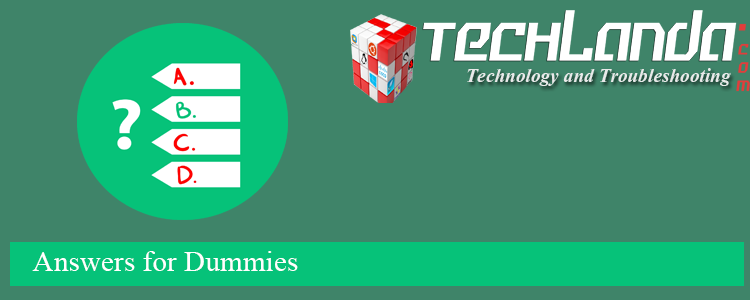# Quiz

## 1024 bits are equal to how many bytes?

### 1024 bits are equal to how many bytes?

• 128 bytes
• 1 byte
• 64 bytes
• 32 bytes## Conversion Definitions

### What is a bit (b)?

A bit is a unit used to measure digital storage and is based on "Binary multiples of bits". The symbol for bit is b. There are 8 bits in a Byte.

### What is a Byte (B)?

A Byte is a unit used to measure digital storage and is based on "Binary multiples of Bytes". The symbol for Byte is B. There are 0.125 Bytes in a bit.

## Conversion Formula

The formula to convert from bits to Bytes is:
`Bytes = bits ÷ 8`

## Conversion Example

### bit to Byte Conversion Example

```Task: Convert 3,000 bits to Bytes (show work)

Formula:
bits ÷ 8 = Bytes

Calculations:
3,000 bits ÷ 8 = 375 Bytes

Result:
3,000 bits is equal to 375 Bytes```